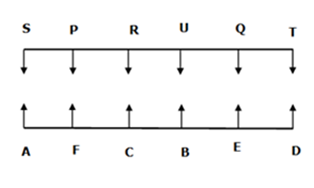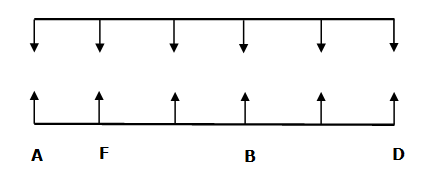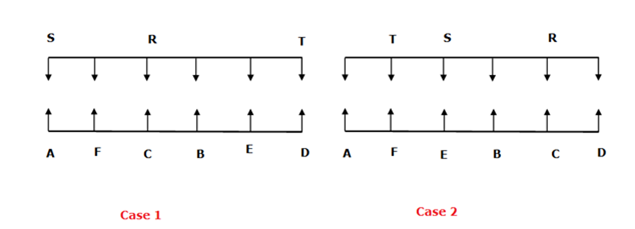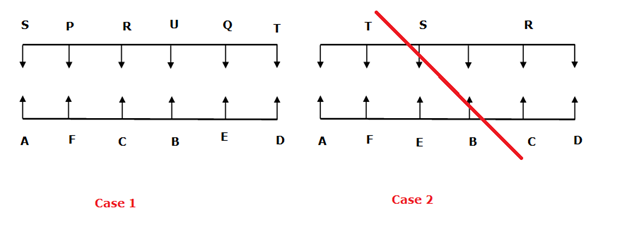# IBPS Clerk Prelims Reasoning (Day-07)

Dear Aspirants, Our IBPS Guide team is providing new series of Reasoning Questions for IBPS Clerk Prelims 2020 so the aspirants can practice it on a daily basis. These questions are framed by our skilled experts after understanding your needs thoroughly. Aspirants can practice these new series questions daily to familiarize with the exact exam pattern and make your preparation effective.

Start Quiz

Time-7 min

Parallel row seating

Direction (1-5): Study the following information carefully and answer the below questions

Twelve persons are sitting in two parallel rows containing 6 people each, in such a way that there is equal distance between adjacent persons. In row-1 P, Q, R, S, T and U are seated (but not necessarily in the same order) and all of them facing south. In row-2 A, B, C, D, E and F are seated (but not necessarily in the same order) and all of them facing north. Therefore in the given seating arrangement, each member seating in the row faces another member of the other row.

B sits second to the right of F who sits fourth to the left of D. A is not an immediate neighbour of B. F is not sit at any extreme end. R faces C. R sits second to the left of S. The one who sits immediate neighbour of T faces E. T is not an immediate neighbour of R. Q sits second to the left R. P sits second to the right of U.

1) Four of them are alike in a certain way and form a group. Which of the following does not belong to the group?

A) F-P

B) R-C

C) S-D

D) U-B

E) Q-E

2) Who among the following sits at the end of the row?

A) TA

B) FR

C) DR

D) SB

E) CP

3) Who sits third to the right of Q?

A) S

B) R

C) T

D) P

E) U

4) Who among the following sits second to the right of the one who faces U?

A) E

B) F

C) P

D) C

E) D

5) Who among the following sits opposite of R?

A) F

B) A

C) B

D) C

E) D

Misc

6) In the word “PRESSURE” all the vowel letters are changed to their previous letters in the alphabetical order, then how many letters are there appear more than one time?

A) None

B) 1

C) 2

D) 3

E) None of these

Inequality

Direction (7-9): In each of the following questions, the relationship between different elements is shown in the statements followed by two conclusions. Find the conclusion which is definitely true.

7) Statements:

E < F > G = H; H > L > M > R

Conclusions:

I). F > R

II). E > M

A) If only conclusion I follows.

B) If only conclusion II follows.

C) If either conclusion I or II follows.

D) If neither conclusion I nor II follows.

E) If both conclusions I and II follow.

8) Statements:

A > F ≥ P ≥ Q ≥ N ; N ≥ L ≥ O ≥ R;

Conclusions:

I). F > R

II). F = R

A) If only conclusion I follows.

B) If only conclusion II follows.

C) If either conclusion I or II follows.

D) If neither conclusion I nor II follows.

E) If both conclusions I and II follow.

9) Statements:

Z < Y > M ≥ P > S; S ≥ R ≤ O

Conclusions:

I). Z < R

II). M > R

A) If only conclusion I follows.

B) If only conclusion II follows.

C) If either conclusion I or II follows.

D) If neither conclusion I nor II follows.

E) If both conclusions I and II follow.

Misc

10) How many such digits are there in the number “8913479256” which remains its original position when all the digits are arranged in descending order from left to right?

A) 1

B) 4

C) 2

D) 3

E) None

Directions (1-5):B sits second to the right of F who sits fourth to the left of D. F is not sit at any extreme end. A is not an immediate neighbour of B.R faces C. R sits second to the left of S. The one who sits immediate neighbour of T faces E. T is not an immediate neighbour of R. From the above conditions, there are two possibilities,Q sits second to the left R. P sits second to the right of U.  From the above condition, Case 2 was dropped and the final arrangement shown in Case1P R E S S U R E

P R D S S T R D => DD, SS, RR

Directions (7-9):